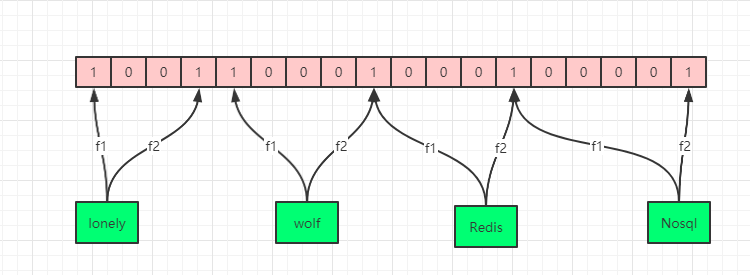# 布隆过滤器以及如何删除

## 布隆过滤器介绍

“实际上就是个位数组，元素经过几个hash函数，分别把位数组对应位置设置为1。判断是否存在这个元素，直接去对应位置判断是不是1。”

### 什么是布隆过滤器1. 如果布隆过滤器判断一个元素存在，那么这个元素可能存在
2. 如果布隆过滤器判断一个元素不存在，那么这个元素一定不存在

## bloom过滤器简单demo

“实际上就是个位数组，元素经过几个hash函数，分别把位数组对应位置设置为1。判断是否存在这个元素，直接去对应位置判断是不是1。”

``````public static class MyBloomFilter
{
private static BitArray _body; //位数组
private static int _size; // 数组长度

public static void Init()
{
_size = 65535;
_body = new BitArray(_size);
}

{
// 三个hash函数
var hash1 = Math.Abs(Hash(str));
var hash2 = Math.Abs(Hash(str + "1"));
var hash3 = Math.Abs(Hash(str + "2"));
// 将对应位设置1
_body[hash1 & _size] = true;
_body[hash2 & _size] = true;
_body[hash3 & _size] = true;
}

private static int Hash(string str)
{
return str.GetHashCode();
}

public static bool Contains(string str)
{
var hash1 = Math.Abs(Hash(str));
var hash2 = Math.Abs(Hash(str + "1"));
var hash3 = Math.Abs(Hash(str + "2"));
return _body[hash1 & _size] && _body[hash2 & _size] && _body[hash3 & _size];
}
}
``````

## bloom过滤器的删除

1byte=8bit，11111111 = 255

“实际上就是个字节数组，元素经过几个hash函数，分别把位数组对应位置+1，判断是否存在这个元素，直接去对应位置判断是不是大于1。删除某个元素就对应位置-1。”

``````public class MyCounterBloomFilter
{
private static byte[] _body; //byte数组
private static int _size; // 数组长度
private static int _sizemask; // 数组长度-1

public static void Init(int size = 65535)
{
if ((size & size - 1) != 0)
{
throw new Exception("数组长度必须为2的幂，求余数用了与运算");
}

_size = size;
_body = new byte[_size];
}

{
// 三个hash函数
var hash1 = Math.Abs(Hash(str));
var hash2 = Math.Abs(Hash(str + "1"));
var hash3 = Math.Abs(Hash(str + "2"));
// 将对应位+1
}

private static int Hash(string str)
{
return str.GetHashCode();
}

public static bool Contains(string str)
{
var hash1 = Math.Abs(Hash(str));
var hash2 = Math.Abs(Hash(str + "1"));
var hash3 = Math.Abs(Hash(str + "2"));
return _body[hash1 & _sizemask] > 0 && _body[hash2 & _sizemask] > 0 && _body[hash3 & _sizemask] > 0;
}

public static void Delete(string str)
{
var hash1 = Math.Abs(Hash(str));
var hash2 = Math.Abs(Hash(str + "1"));
var hash3 = Math.Abs(Hash(str + "2"));